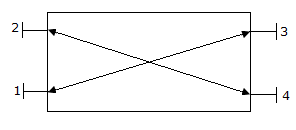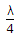# Electronics and Communication Engineering - Microwave Communication

### Exercise :: Microwave Communication - Section 6

11.

Slotted line can be used for measurement of high frequencies

 A. True B. False

Answer: Option A

Explanation:

No answer description available for this question. Let us discuss.

12.

In a waveguide the dielectric region is generally

 A. air B. magnetic material C. brass D. mica

Answer: Option A

Explanation:

No answer description available for this question. Let us discuss.

13.

In directional coupler of the given figure, the terms |S14| and |S23| of scattering matrix are nearlyA. 1 each B. 0.5 each C. 0.1 each D. 1 and 0.5 respectively

Answer: Option A

Explanation:

No answer description available for this question. Let us discuss.

14.

A line is excited by a 100 V, 200 ohm source and is terminated by 100 Ω resistance. If the voltage on line reaches steady value in 80 ns, the natural resonant frequency is

 A. 100 MHz B. 50 MHz C. 12.5 MHz D. 6.25 MHz

Answer: Option C

Explanation:

No answer description available for this question. Let us discuss.

15.

A line of length slightly longer thanand open circuited at far end behaves as

 A. inductance L B. capacitance C C. L and C in series D. L and C in parallel

Answer: Option A

Explanation:

No answer description available for this question. Let us discuss.

#### Current Affairs 2021

Interview Questions and Answers